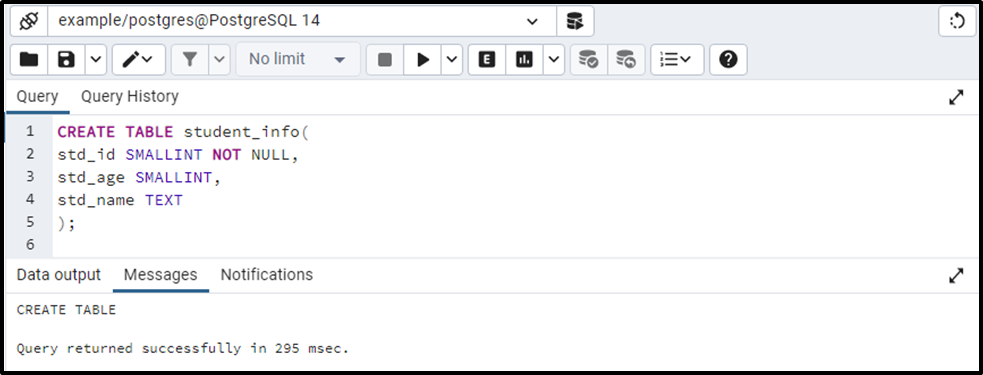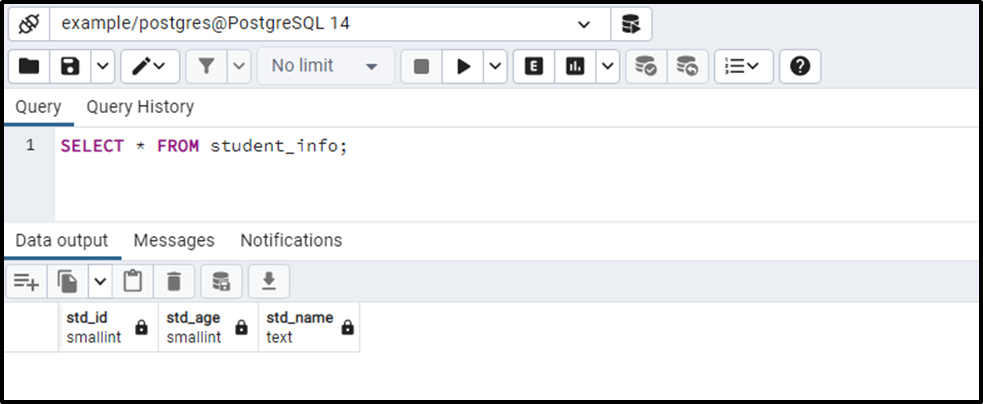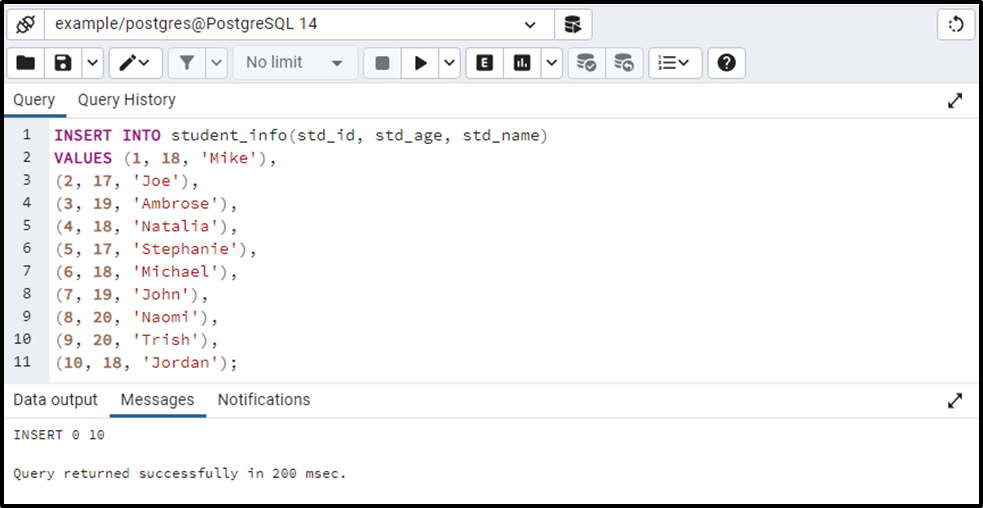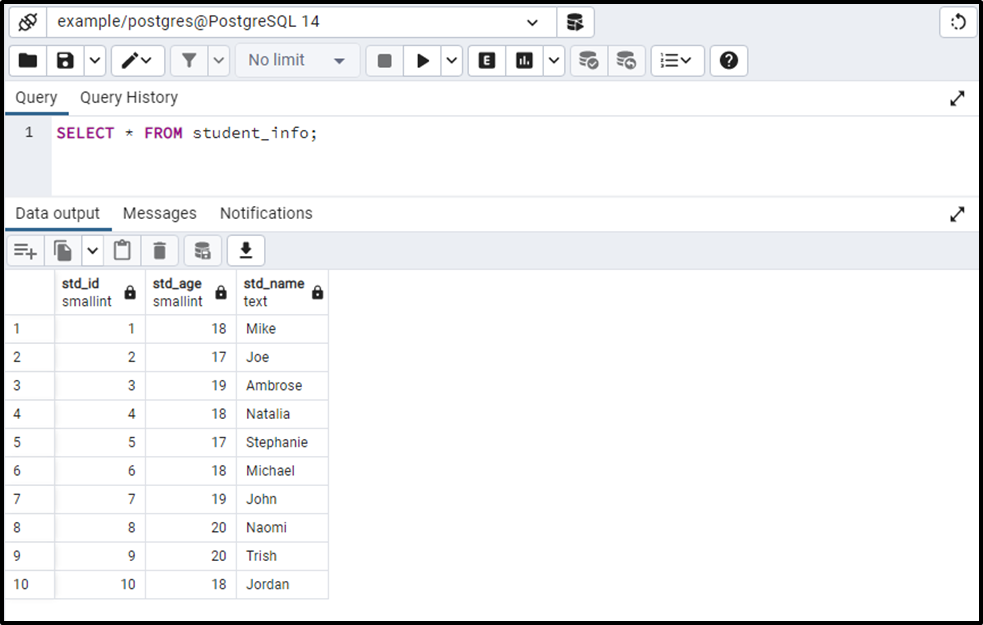##### PostgreSQL SMALLINT Data Type With Examples

PostgreSQL offers different data types to deal with integer values, SMALLINT is one of them. A SMALLINT data type takes two bytes of storage and can store integers between -32,768 and +32,768. It is used to store small values like someone's age, the number of pages in a book, etc.

So, let’s learn how the SMALLINT data type works with the help of examples.

### Why SMALLINT?

The below-listed points describe the need for the SMALLINT data type:

- The INTEGER/INT is a data type commonly used data type in PostgreSQL to store the integers. However, If we have to store the small numeric values like people’s age, then we can use the SMALLINT data type instead of the INTEGER data type.

- The SMALLINT takes a minimum storage size to store a value. So, it is preferred that if you have a small numeric value, then use the SMALLINT data type.

- If the value to be stored exceeds the limit of the SMALLINT data type, then use the INTEGER data type.

- The BIGINT data type should be used only if the value to be stored doesn’t fit in the range of INTEGER data type.

Note: PostgreSQL will throw an error if you insert an out-of-range value.

### How to Use SMALLINT in Postgres?

Let’s consider a couple of examples to understand how the SMALLINT data type works in PostgreSQL.

### Example #1: Create a Table Having SMALLINT Data Type

Here is the code to create a column with SMALLINT data type:

```CREATE TABLE student_info(
std_id SMALLINT NOT NULL,
std_age SMALLINT,
std_name TEXT
);```

Here, we created a table named student_info that consists of three columns: std_id, std_age, and std_name. The data type of std_id and std_age columns is SMALLINT, while std_name has a TEXT data type:The above snippet shows that the table named student_info has been created successfully. Let’s verify the table’s creation using the SELECT query:

`SELECT * FROM student_info;`The table named student_info with three columns has been created successfully.

### Example #2: Insert SMALLINT Data Into Student_info Table

Let’s execute the INSERT INTO command to insert data into the student_info table:

```INSERT INTO student_info(std_id, std_age, std_name)
VALUES (1, 18, 'Mike'),
(2, 17, 'Joe'),
(3, 19, 'Ambrose'),
(4, 18, 'Natalia'),
(5, 17, 'Stephanie'),
(6, 18, 'Michael'),
(7, 19, 'John'),
(8, 20, 'Naomi'),
(9, 20, 'Trish'),
(10, 18, 'Jordan');```Ten records have been inserted into the student_info table successfully. Let’s execute the SELECT statement to check the newly inserted data:

`SELECT * FROM student_info;`All the records have been inserted into the student_info table successfully.

That was all the basics you should learn before starting with the SMALLINT data type.

### Conclusion

In PostgreSQL, the SMALLINT is one of the integer data types that is used to store small integer values. The SMALLINT data type takes only two bytes of storage and can store integers between -32,768 to +32,768. Using some examples, this write-up demonstrated what SMALLINT is, what is the need for SMALLINT data type, and how to use it in PostgreSQL.